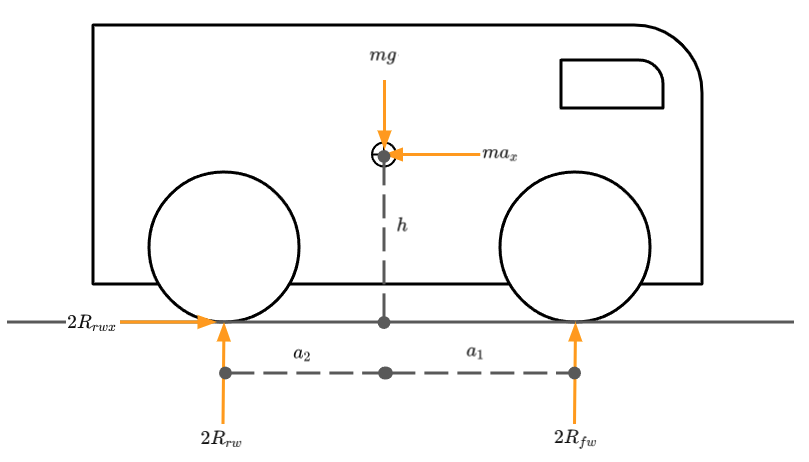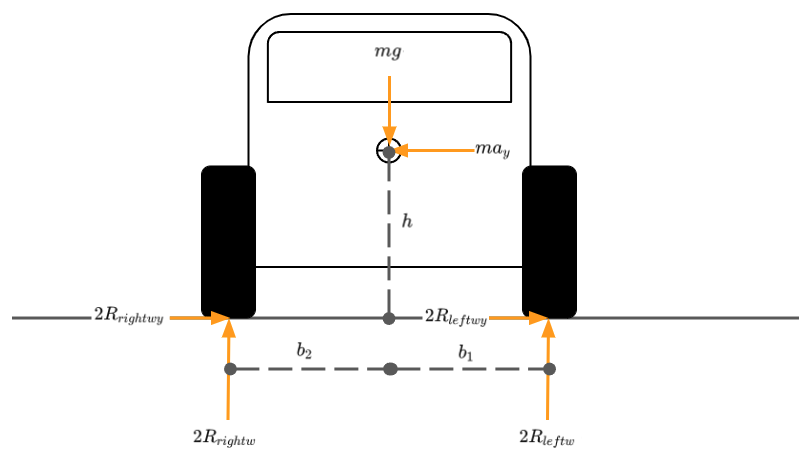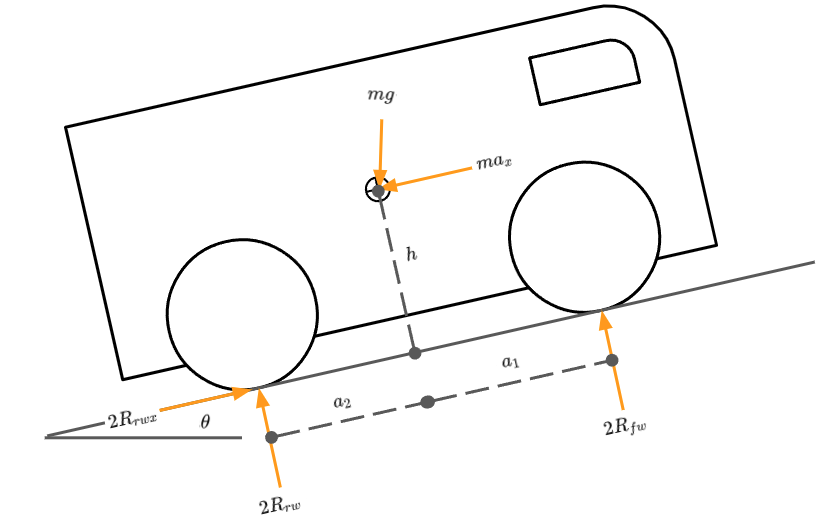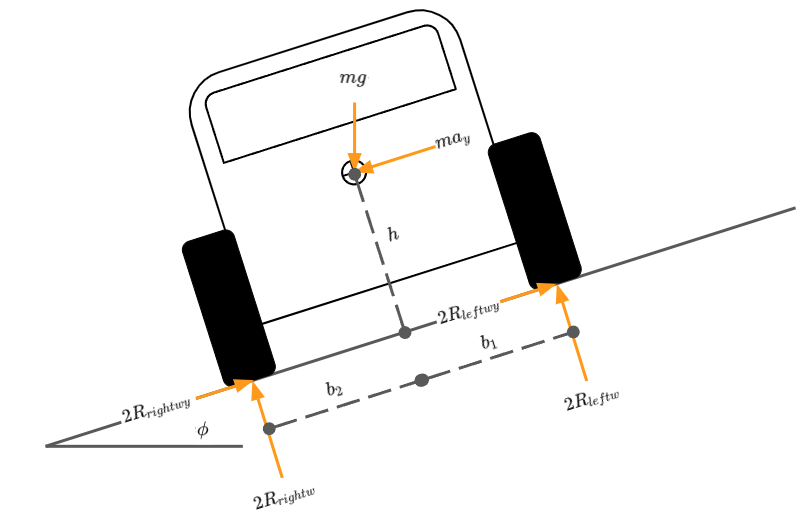# Weight Distribution in Mobile Ground Vehicles

## Preamble

A ground vehicle’s motion is generally only ever limited by one of two things, torque or friction. There are many ways to try and maximise both of these. This post will look to address friction by considering the normal forces at the contact patches between the wheels and the ground.

When designing a mobile ground vehicle, weight distribution is one area that can be optimised fairly freely to provide large benefits. These advantages in some cases can be gained with no need for additional parts or systems, rather, by positioning components that are already present in a more appropriate way.

I will examine the case for a four wheeled, rear drive mobile robotic platform but the models presented here are easily modified for more or fewer wheels. I will start out by presenting a model showing key design parameters, followed by some practical applications of the model. Detailing a method for measurement/ validation of the key parameters on a real ground vehicle, tilting angles, working out the maximum operable incline and also modeling for the case of compound pitch and roll angles. Finally, I’ve given some Python code to run through all of this to help get things started.

Please note that this post is designed as an introductory primer to this very deep topic. Some great books on the subject are provided in the Resources and Further Reading section at the end of the post.

## Model

To get a better understanding we first need a framework for the key design parameters that relate to the normal forces at the wheels. Why do we care about the reactions at the wheels?

The normal forces are directly proportional to the amount of lateral and longitudinal force each wheel can generate for accelerating, breaking or cornering ie. the useful work that we can get out of a wheel. The key variables we have to play with are the normal force, $R_{rw}$, and the friction coefficient, $\mu$. If we look at the longitudinal force, $R_{rwx}$.

$\begin{equation} R_{rw} = \mu R_{rwx} \tag{1} \end{equation}$

Usually we cannot do a great deal to alter the friction coefficient of the ground surface, especially when dealing with outdoor scenarios. We do however have control over the vehicle’s wheel’s friction coefficient, as well as the centre of mass location of the vehicle, which plays a key role in the normal force. Increasing the values of $\mu$ and $R_{rw}$ allow us to get more work out each wheel with fewer slip losses. There is also the nice side benefit of increased odometry accuracy.

### Level Ground Side View

Let’s start looking at our vehicle from the side in the $xz$ plane. Figure 1 shows our vehicle driving forwards from left to right. We are defining $x$ right as positive, $z$ down as positive and moments about the $y$ axis clockwise positive.Figure 1: Level Ground Side View Free Body Diagram

The vehicle’s wheelbase can be defined as $l$ as shown in equation (2). $\begin{equation} l = a_1 + a_2 \tag{2} \end{equation}$

Working in figure 1 we can apply the Newtonian laws of motion to this free body problem.

Working in the $x$ direction. $\begin{equation} \sum F_x = 0 \tag{3} \end{equation}$

$\begin{equation} \sum F_x = 2R_{rwx} - ma_x = 0 \tag{4} \end{equation}$

Working in the $z$ direction. $\begin{equation} \sum F_z = 0 \tag{5} \end{equation}$

$\begin{equation} \sum F_z = mg - 2R_{rw} - 2R_{fw} = 0 \tag{6} \end{equation}$

Summing moments at the centre of gravity about the $y$ axis. $\begin{equation} \sum M_{yy} = 0 \tag{7} \end{equation}$

$\begin{equation} \sum M_{yy} = 2 a_2 R_{rw} - 2 h R_{rwx} - 2 a_1 R_{fw} = 0 \tag{8} \end{equation}$

Or at the rear wheel contact patch.

$\begin{equation} \sum M_{yy} = a_2 m g - 2 l R_{fw} - h m a_x = 0 \tag{9} \end{equation}$

Taking the above equations, we can then solve for our three unknown reactions $R_{fw}$, $R_{rw}$ and $R_{rwx}$. Doing so yields equations (10), (11) and (12).

$\begin{equation} R_{rwx} = \frac {1}{2} ma_x \tag{10} \end{equation}$

$\begin{equation} R_{fw} = \frac {1}{2} mg \frac {a_2}{l} - \frac {1}{2} ma_x \frac {h}{l} \tag{11} \end{equation}$

$\begin{equation} R_{rw} = \frac {1}{2} mg \frac {a_1}{l} + \frac {1}{2} ma_x \frac {h}{l} \tag{12} \end{equation}$

When our ground vehicle is not accelerating, $a_x$ equals zero, the conditions are met for static equilibrium. This results in equations (11) and (12) breaking into two parts. A static contribution, either $\frac {1}{2} mg \frac {a_1}{l}$ or $\frac {1}{2} mg \frac {a_2}{l}$, and a dynamic contribution $\frac {1}{2} ma_x \frac {h}{l}$.

### Level Ground Front View

The exact same process is applicable for the front view, $yz$ plane, of the vehicle. Figure 2 shows our vehicle driving forwards towards the viewer. We are defining $y$ right for the vehicle as positive (left in the image), $z$ down as positive and moments about the $x$ axis counter-clockwise positive.Figure 2: Level Ground Front View Free Body Diagram

The vehicle’s track can be defined as $t$ as shown in equation (13). $\begin{equation} t = b_1 + b_2 \tag{13} \end{equation}$

Working in figure 2 we can apply the Newtonian laws of motion to this free body problem.

Working in the $y$ direction. $\begin{equation} \sum F_y = 0 \tag{14} \end{equation}$

$\begin{equation} \sum F_y = ma_y - 2R_{leftwy} - 2R_{rightwy} \tag{15} \end{equation}$

Working in the $z$ direction. $\begin{equation} \sum F_z = 0 \tag{16} \end{equation}$

$\begin{equation} \sum F_z = mg - 2R_{leftw} - 2R_{rightw} \tag{17} \end{equation}$

Summing moments at the centre of gravity about the $x$ axis. $\begin{equation} \sum M_{xx} = 0 \tag{18} \end{equation}$

$\begin{equation} \sum M_{xx} = 2 h (R_{leftw} + R_{rightw}) + 2 b_1 R_{leftw} - 2 b_2 R_{rightw} = 0 \tag{19} \end{equation}$

Summing moments at the right contact patch. $\begin{equation} \sum M_{xx} = ma_y h - m_g b_2 + 2 R_{leftw} = 0 \tag{20} \end{equation}$

Taking the above equations we can return normal force contributions for the wheels on the left and right sides of the vehicle. $\begin{equation} R_{leftw} = \frac {1}{2} mg \frac {b_2}{t} - \frac {1}{2} ma_y \frac {h}{t} \tag{21} \end{equation}$

$\begin{equation} R_{rightw} = \frac {1}{2} mg \frac {b_1}{t} + \frac {1}{2} ma_y \frac {h}{t} \tag{22} \end{equation}$

As with the side view case these equations can be split into their static contribution $\frac {1}{2} mg \frac {b_1}{t}$ or $\frac {1}{2} mg \frac {b_2}{t}$ and dynamic contribution $\frac {1}{2} ma_y \frac {h}{t}$.

### Ascending Incline

We can take the case depicted in figure 1 and model the effect of the vehicle ascending an inclination. This is shown in figure 3.Figure 3: Incline Side View Free Body Diagram

$\begin{equation} R_{fw} = \frac {1}{2} mg (cos \theta \frac {a_2}{l} - sin \theta \frac {h}{l}) - \frac {1}{2} ma_x \frac {h}{l} \tag{23} \end{equation}$

$\begin{equation} R_{rw} = \frac {1}{2} mg (cos \theta \frac {a_1}{l} + sin \theta \frac {h}{l}) + \frac {1}{2} ma_x \frac {h}{l} \tag{24} \end{equation}$

$\begin{equation} R_{rwx} = \frac {1}{2} mg sin \theta \tag{25} \end{equation}$

### Traversing Incline

Similarly to the previous scenario, we can take the case depicted in figure 2 and model the effect of the vehicle traversing along an inclination. This is shown in figure 4.Figure 4: Incline Front View Free Body Diagram

$\begin{equation} R_{leftw} = \frac {1}{2} mg (cos \phi \frac {b_2}{t} - sin \phi \frac {h}{t}) - \frac {1}{2} ma_y \frac {h}{t} \tag{26} \end{equation}$

$\begin{equation} R_{rightw} = \frac {1}{2} mg (cos \phi \frac {b_1}{t} + sin \phi \frac {h}{t}) + \frac {1}{2} ma_y \frac {h}{t} \tag{27} \end{equation}$

## Applications

All this theory and text book style free-body diagrams are all well and good but what can we actually do with this?

### Real Vehicle Design Validation

I’ll now present a simple method for validating that our design intent is what we have present in our real ground vehicle. This method is useful for prototype design validation, production design validation or even reverse engineering other vehicles.

#### Level Ground Corner Weights

With four sets of weighing scales and the ground vehicle on level ground, we can measure the weight of the vehicle at each of the four wheel’s contact patches. We can use these measurements in tandem with the model presented earlier for the level ground reactions in equations (11), (12), (21) and (22). With some re-arrangement of those equations and dropping the dynamic contributions, we can derive the centre of mass location for our real ground vehicle from the measured corner weights.

$\begin{equation} m_f = R_{fw}g \tag{28} \end{equation}$

$\begin{equation} m_r = R_{rw}g \tag{29} \end{equation}$

Working in the side view, $xz$ plane. $\begin{equation} a_1 = \frac {2R_{rearw}}{m_{tot}g}l \end{equation}$

$\begin{equation} a_2 = \frac {2R_{frontw}}{m_{tot}g}l \end{equation}$

Working in the front view, $yz$ plane. $\begin{equation} b_1 = \frac {2R_{rightw}}{m_{tot}g}t \end{equation}$

$\begin{equation} b_2 = \frac {2R_{leftw}}{m_{tot}g}t \end{equation}$

#### Inclination Test

A similar process can be carried out for determining the height of the centre of mass by tilting the vehicle to a known inclination angle, effectively recreating the conditions in 3. We can either take the mass under both rear wheels or both front wheels, however as the scales will be perpendicular to the gravity vector we now need a new equation that also takes into account the wheel radii.

##### TODO: Add image and rear wheel equation

Raised height of front wheel $= h_{frontw}$ m

Inclination angle $= \sin(\frac {h_{frontw}}{l})$ degrees

Mass under front wheels $m_{faxle}$ kg

Front wheel radius $= rad_f$ m

Rear wheel radius $= rad_r$ m

We can calculate the centre of mas height as follows.

$\begin{equation} h = rad_r + (cog2r - m_{faxle} / m * l) * \cot \theta - m_{faxle} / m * (rad_r - rad_f) \end{equation}$

For a more accurate representation try multiple inclination angles and take the median or mean.

### Tilting Angles

Given the centre of mass position it is possible to determine the angles at which a ground vehicle would roll over. This condition occurs when the gravity vector falls outside of the polygon drawn by the contact patches of the vehicle’s wheels.

$\begin{equation} \theta_{t_{front}} = atan(\frac {a_1}{h}) \tag{30} \end{equation}$

$\begin{equation} \theta_{t_{rear}} = atan(\frac {a_2}{h}) \tag{31} \end{equation}$

$\begin{equation} \theta_{t_{left}} = atan(\frac {b_1}{h}) \tag{32} \end{equation}$

$\begin{equation} \theta_{t_{right}} = atan(\frac {b_2}{h}) \tag{33} \end{equation}$

### Maximum Operable Incline

Whereas the tilting angles previously shown provide when the vehicle will roll over, in most real cases a vehicle will slide before it rolls. We can determine the angles at which the vehicle will begin to slide if we have an estimate for the friction coefficient between the vehicle’s wheels and the ground surface.

Assuming drive and breaking occur at the rear wheels only.

Ascending an incline $\begin{equation} \theta = acot ( \frac {l}{\mu a_1} - \frac {h}{a_1}) \end{equation}$

Descending an incline $\begin{equation} \theta = acot ( \frac {l}{- \mu a_1} - \frac {h}{a_1}) \end{equation}$

### Modelling the Complex Case

#### Normal Forces

It is usually a fair assumption that all of the longitudinal weight transfer occurs equally between the left and right side of the vehicle. This is given that most vehicles tend to be approximately structurally symmetric, in terms of stiffness between the left and right sides of the vehicle.

This assumption however often doesn’t hold for the lateral weight transfer that occurs between the front axle and rear axle, as most vehicles are asymmetric between the front and rear. As such it can be useful to define a constant to define this with values ranging between 0 and 1:

Portion of Lateral Weight Transfer Occurring at the Front Axle = $\rho_{mf}$

Note that $\rho_{mf}$ = 0.5 would imply structural symmetry between the front and rear of the vehicle.

$\begin{equation} R_{fl} = m g (\cos \theta \frac {a_2}{l} - \sin \theta \frac {h}{l}) (\cos \phi \frac {b_2}{t} - \sin \phi \frac {h}{t}) - \frac {1}{2} m a_x \frac {h}{l} - \rho_{mf} m a_y \frac {h}{t} \end{equation}$

$\begin{equation} R_{fr} = m g (\cos \theta \frac {a_2}{l} - \sin \theta \frac {h}{l}) (\cos \phi \frac {b_1}{t} + \sin \phi \frac {h}{t}) - \frac {1}{2} m a_x \frac {h}{l} + \rho_{mf} m a_y \frac {h}{t} \end{equation}$

$\begin{equation} R_{rl} = m g (\cos \theta \frac {a_1}{l} + \sin \theta \frac {h}{l}) (\cos \phi \frac {b_2}{t} - \sin \phi \frac {h}{t}) + \frac {1}{2} m a_x \frac {h}{l} - (1 - \rho_{mf}) m a_y \frac {h}{t} \end{equation}$

$\begin{equation} R_{rr} = m g (\cos \theta \frac {a_1}{l} + \sin \theta \frac {h}{l}) (\cos \phi \frac {b_1}{t} + \sin \phi \frac {h}{t}) + \frac {1}{2} m a_x \frac {h}{l} + (1 - \rho_{mf}) m a_y \frac {h}{t} \end{equation}$

### Python Implementation

Let’s start by importing Numpy and defining some functions. I’ve short handed several trigonometric functions for brevity. I have also created two custom functions, one which compiles a 3D rotation matrix from Euler angles, and a second function that takes a slopes inclination and the attack angle of the vehicle to that inclination and returns the vehicle’s pitch and roll angles.

import numpy as np

# Short handing trig functions
sin = np.sin
cos = np.cos
tan = np.tan
asin = np.arcsin
acos = np.arccos
atan = np.arctan
atan2 = np.arctan2
pi = np.pi
to_degs = 180/pi

def cot(angle):
"""Calculate the cotangent of an angle
"""
out = 1/tan(angle)
return out

def acot(angle):
"""Calculate the arc cotangent of an angle
"""
out = atan(1/angle)
return out

def hpr2mat_parent2child(hea, pit, rol):
'''Takes three Euler angles and returns a parent
to child frame rotation matrix
'''
[0, 0, 1]])

[0, 1, 0],

cc = np.array([[1, 0, 0],

if hea != 0 and pit == 0 and rol == 0:
return ca

if hea == 0 and pit != 0 and rol == 0:
return cb

if hea == 0 and pit == 0 and rol != 0:
return cc

rot = ca @ cb @ cc

rot = np.linalg.inv(rot)

return rot

def calculate_orientation(attack_angle, incline):
'''Takes an attack angle and inclination angle and
returns vehcile pitch and roll angles
'''
compound_rot = hpr2mat_parent2child(attack_angle, incline, 0)

x_unit = np.array([1, 0, 0])
y_unit = np.array([0, 1, 0])

x_rot = compound_rot @ x_unit
x_rot_proj = np.array([x_rot, x_rot, 0])

y_rot = compound_rot @ y_unit
y_rot_proj = np.array([y_rot, y_rot, 0])

pitch = acos((x_rot.T @ x_rot_proj) / (np.linalg.norm(x_rot) * np.linalg.norm(x_rot_proj))) * to_degs
roll = acos((y_rot.T @ y_rot_proj) / (np.linalg.norm(y_rot) * np.linalg.norm(y_rot_proj))) * to_degs

return pitch, roll

Next we can call these functions in order to establish the pitch and roll of the vehicle on a given incline.

attack_angle = 45
incline = 5

pitch, roll = calculate_orientation(attack_angle, incline)

Here I have given an attack angle of 45 degrees and an incline angle of 5 degrees, which results in a vehicle pitch of 3.533 degrees and a roll of 3.533 degrees.

Now we can specify all of our vehicle’s key parameters, the variable names ought to match those mentioned earlier in this post for clarity.

# Vehicle parameters
m = 40 # kg

# Vehicle CoG longitudinal position
a_1 = 0.4 # m
a_2 = 0.3 # m
l = a_1 + a_2

# Vehicle CoG lateral position
b_1 = 0.9/2 # m
b_2 = 0.9/2 # m
t = b_1 + b_2

# Vehicle CoG vertical position
h = 0.4 # m

# Proportion of Lateral Weight Transfer at the Front of the Vehicle
rho_mf = 0.5

# Friction Coefficient
mu = 0.6

We also need to add the dynamic boundary conditions and accelerations present at the given moment in time we wish to model.

# Dynamic conditions
g = 9.81 # m^2 / s

forward_speed = 0.4 # m / s
corner_radius = 15 # m | Positive for turning left

a_x = 3 # m^2 / s | Positive for forward acceleration
a_y = forward_speed**2 / corner_radius # m^2 / s | Positive for turning left
# Compute tilting Angles
theta_t_front = round(atan2(a_1, h) * to_degs, 2)
theta_t_rear = round(atan2(a_2, h) * to_degs, 2)
theta_t_left = round(atan2(b_1, h) * to_degs, 2)
theta_t_right = round(atan2(b_2, h) * to_degs, 2)

# Compute the maximum operable inclines before slipping
max_asc_angle = round(acot(l/(mu * a_1) - h/a_1) * to_degs, 2)
max_des_angle = round(acot(l/(-mu * a_1) - h/a_1) * to_degs, 2)

# TODO: add max traversing angle

# Debug values
static_front_axle = m * g * (cos(pitch) * a_2 / l - sin(pitch) * h / l)
static_rear_axle = m * g * (cos(pitch) * a_1 / l + sin(pitch) * h / l)

static_left_side = m * g * (cos(roll) * b_2 / t - sin(roll) * h / t)
static_right_side = m * g * (cos(roll) * b_1 / t + sin(roll) * h / t)

dynamic_longitudinal_transfer = m * a_x * h / l
dynamic_lateral_transfer = m * a_y * h / t

Now we can compute the normal forces under all four wheels and the subsequent masses that you might measure if you could put scales under those wheels.

# Compute reaction forces
R_fl = m * g * (cos(pitch) * a_2 / l - sin(pitch) * h / l) * (cos(roll) * b_2 / t - sin(roll) * h / t) - 1/2 * m * a_x * h / l - rho_mf * m * a_y * h / t
R_fr = m * g * (cos(pitch) * a_2 / l - sin(pitch) * h / l) * (cos(roll) * b_1 / t + sin(roll) * h / t) - 1/2 * m * a_x * h / l + rho_mf * m * a_y * h / t
R_rl = m * g * (cos(pitch) * a_1 / l + sin(pitch) * h / l) * (cos(roll) * b_2 / t - sin(roll) * h / t) + 1/2 * m * a_x * h / l - (1 - rho_mf) * m * a_y * h / t
R_rr = m * g * (cos(pitch) * a_1 / l + sin(pitch) * h / l) * (cos(roll) * b_1 / t + sin(roll) * h / t) + 1/2 * m * a_x * h / l + (1 - rho_mf) * m * a_y * h / t

R_fl = round(R_fl, 3)
R_fr = round(R_fr, 3)
R_rl = round(R_rl, 3)
R_rr = round(R_rr, 3)

# Compute corner weights
m_fl = round(R_fl / g, 3)
m_fr = round(R_fr / g, 3)
m_rl = round(R_rl / g, 3)
m_rr = round(R_rr / g, 3)

Our inputs for the vehicle and conditions yield the following…

Static Conditions | Pitch ($\theta$) = 3.533 degrees | Roll ($\phi$) = 3.533 degrees

Dynamic Conditions | Forward Acceleration ($a_x$) = 3 $m/s^2$ | Lateral Acceleration ($a_y$) = 0.0106667 $m/s^2$

Under the Front Left Wheel | Mass = 3.901 kg | Normal Force = 38.271 N

Under the Front Right Wheel | Mass = 4.781 kg | Normal Force = 46.898 N

Under the Rear Left Wheel | Mass = 14.91 kg | Normal Force = 146.267 N

Under the Rear Right Wheel | Mass = 16.256 kg | Normal Force = 159.474 N

Sum of Calculated Masses = 39.848 kg | Original Mass = 40 kg

# Hey you!

### Found this useful or interesting?

Surely after all this I deserve a drink?! Consider donating to support.### Any question, comments, corrections or suggestions?

Reach out on the social links below through the buttons.